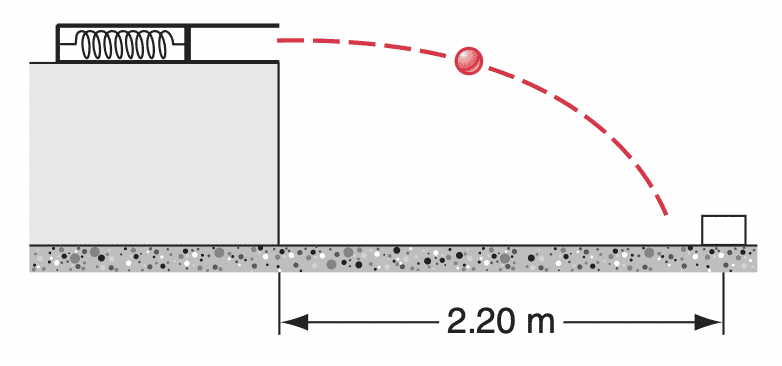# Springs and Potential Energy

• jolly_math
In summary, the problem states that the gun is mounted on a table and that the target is on the floor. You don't need the height of the table to solve this problem. I strongly suggest that you adopt an algebraic approach, i.e. use symbols and not numbers when you write equations. Use symbols for unknown quantities and don't worry if you don't have numbers for them. It will all come out in the wash.

#### jolly_math

Homework Statement
Two children are playing a game in which they try to hit a small box on the floor with a marble fired from a spring-loaded gun that is mounted on a table. The target box is 2.20 m horizontally from the edge of the table. Bobby compresses the spring 1.10 cm, but the marble falls 27.0 cm short. How far should Rhoda compress the spring to score a hit?
Relevant Equations
U(x) = (1/2)kx^2
KE = (1/2)mv^2
Ki + Ui = Kf + Uf
1/2)kx2 = (1/2)mvf2, but W = (1/2)mvf2 = F∆d, so
1/2)kx^2 = F∆d.

The solution says that I should just substitute v as d/t. But could anyone explain why my reasoning is wrong? Thanks.

Which force is this that does the work W = FΔd? What is the direction of F relative to the direction of the displacement Δd? This is a projectile motion problem where you need to find the initial speed of the projectile needed to hit the target. Then you must match that speed to the spring compression.

•topsquark and Lnewqban
So, I'm taking it that the spring gun must be horizontal, and the target has no vertical dimension ( physical size)?

Last edited:
erobz said:
So, I'm taking it that the spring gun must be horizontal, and the target has no vertical dimension?This is the diagram. I just assumed there was no vertical dimension, as the solution didn't consider it and I'm not sure how to calculate it otherwise.

jolly_math said:
View attachment 316004
This is the diagram. I just assumed there was no vertical dimension, as the solution didn't consider it and I'm not sure how to calculate it otherwise.
That is the only configuration I could find a solution for with the information given.

Have you worked it out the approach yet?

jolly_math said:
View attachment 316004
This is the diagram. I just assumed there was no vertical dimension, as the solution didn't consider it and I'm not sure how to calculate it otherwise.
The problem states that the gun is mounted on a table and that the target is on the floor. You don't need the height of the table to solve this problem. I strongly suggest that you adopt an algebraic approach, i.e. use symbols and not numbers when you write equations. Don't worry if you don't have numbers for the symbols. It will all come out in the wash.

kuruman said:
The problem states that the gun is mounted on a table and that the target is on the floor. You don't need the height of the table to solve this problem. I strongly suggest that you adopt an algebraic approach, i.e. use symbols and not numbers when you write equations. Use symbols for unknown quantities and don't worry if you don't have numbers for them. It will all come out in the wash.
I was just indicating that a diagram wasn't posted. It was my lack of being able to find a solution for some arbitrary gun angle that led me to conclude that it must be this way.

You don't need the height of the table to solve this problem.
I was talking about the height of the target, as in its physical dimensions. Not where it is located spatially. That was probably confusing, but the OP seemed to understand what I was asking.

Anyhow, It sounds as though the @jolly_math has figured it out.